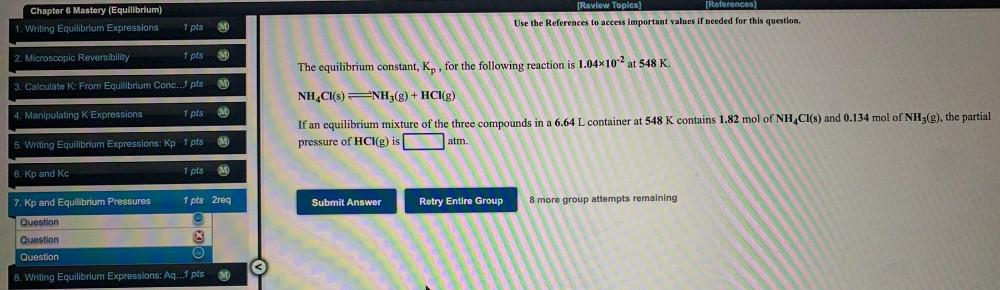Question:

# Chapter 6 Mastery (Equilibrium) 1. Writing Equilibrium Expressions [Review Topics] [References] Use the References to access impChapter 6 Mastery (Equilibrium) 1. Writing Equilibrium Expressions [Review Topics] [References] Use the References to access important values if needed for this question. 1 pts 2. Microscopic Reversibility 1 pts The equilibrium constant, Kp, for the following reaction is 1.04x10-2 at 548 K. 3. Calculate K: From Equilibrium Conc...1 pts (M) NH4CI(S) NH3(g) + HCl(g) 4. Manipulating K Expressions 1 pts M If an equilibrium mixture of the three compounds in a 6.64 L container at 548 K contains 1.82 mol of NH4CI(S) and 0.134 mol of NH3(g), the partial pressure of HCl(g) is atm. M 5. Writing Equilibrium Expressions: Kp 1 pts 6. Kp and kc 1 pts 7. Kp and Equilibrium Pressures 1 pts 2req Submit Answer Retry Entire Group 8 more group attempts remaining Question Question Question 8. Writing Equilibrium Expressions: Ag...1 pts